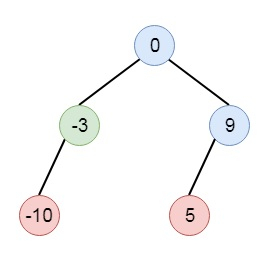# Program to convert a linked list into a binary search tree in C++

Suppose we have a singly linked list where elements are arranged in non-decreasing order, we have to convert it to a height balanced binary search tree. So if the list is like [-10, -3, 0, 5, 9], The possible tree will be like −To solve this, we will follow these steps −

• If the list is empty, then
• return null
• Define a recursive method called sortedListToBST() this will take list start node
• x := address of the previous node of mid node from list a
• mid := exact mid node
• create a new node with value by taking from value of mid
• nextStart := next of mid node
• set mid next as null
• right of node := sortedListToBST(nextStart)
• if x is not null, then
• next of x = null and left of node := sortedListToBST(a)
• return node

Let us see the following implementation to get better understanding −

## Example

Live Demo

#include <bits/stdc++.h>
using namespace std;
class ListNode{
public:
int val;
ListNode *next;
ListNode(int data){
val = data;
next = NULL;
}
};
ListNode *make_list(vector<int> v){
for(int i = 1; i<v.size(); i++){
while(ptr->next != NULL){
ptr = ptr->next;
}
ptr->next = new ListNode(v[i]);
}
}
class TreeNode{
public:
int val;
TreeNode *left, *right;
TreeNode(int data){
val = data;
left = right = NULL;
}
};
void inord(TreeNode *root){
if(root != NULL){
inord(root->left);
cout << root->val << " ";
inord(root->right);
}
}
class Solution {
public:
pair <ListNode*, ListNode*> getMid(ListNode* a){
ListNode* prev = NULL;
ListNode* fast = a;
ListNode* slow = a;
while(fast && fast->next){
fast = fast->next->next;
prev = slow;
slow = slow->next;
}
return {prev, slow};
}
TreeNode* sortedListToBST(ListNode* a) {
if(!a)return NULL;
pair<ListNode*, ListNode*> x = getMid(a);
ListNode* mid = x.second;
TreeNode* Node = new TreeNode(mid->val);
ListNode* nextStart = mid->next;
mid->next = NULL;
Node->right = sortedListToBST(nextStart);
if(x.first){
x.first->next = NULL;
Node->left = sortedListToBST(a);
}
return Node;
}
};
main(){
vector<int> v = {-10,-3,0,5,9};
}
[-10,-3,0,5,9]
-10 -3 0 5 9